## Example Questions

← Previous 1

### Example Question #10 : Solid Geometry

What is the surface area of a cube with a volume of 1728 in3?

144 in2

1728 in2

72 in2

432 in2

864 in2

864 in2

Explanation:

This problem is relatively simple. We know that the volume of a cube is equal to s3, where s is the length of a given side of the cube. Therefore, to find our dimensions, we merely have to solve s3 = 1728. Taking the cubed root, we get s = 12.

Since the sides of a cube are all the same, the surface area of the cube is equal to 6 times the area of one face. For our dimensions, one face has an area of 12 * 12 or 144 in2. Therefore, the total surface area is 6 * 144 = 864 in2.

### Example Question #1 : How To Find The Surface Area Of A Cube

A room has dimensions of 18ft by 15ft by 9ft. The last dimension is the height of the room. It has one door that is 3ft by 7ft and two windows, each 2ft by 5ft. There is no trim to the floor, wall, doors, or windows. What is the total exposed wall space?

1093ft2

553 ft2

1134ft2

594 ft2

2389ft2

553 ft2

Explanation:

If broken down into parts, this is an easy problem. It is first necessary to isolate the dimensions of the walls. If the room is 9 ft high, we know 18 x 15 designates the area of the floor and ceiling. Based on this, we know that the room has the following dimensions for the walls: 18 x 9 and 15 x 9. Since there are two of each, we can calculate the total area of walls - ignoring doors and windows - by doubling the sum of these two areas:

2 * (18 * 9 + 15 * 9) = 2 * (162 + 135) = 2 * 297 = 594 ft2

Now, we merely need to calculate the area "taken out" of the walls:

For the door: 3 * 7 = 21 ft2

For the windows: 2 * (2 * 5) = 20 ft2

The total wall space is therefore: 594 – 21 – 20 = 553 ft2

### Example Question #701 : Sat Mathematics

A certain cube has a side length of 25 m.  How many square tiles, each with an area of 5 m2, are needed to fully cover the surface of the cube?

500

750

200

100

1000

750

Explanation:

A cube with a side length of 25m has a surface area of:

25m * 25m * 6 = 3,750 m2

(The surface area of a cube is equal to the area of one face of the cube multiplied by 6 sides. In other words, if the side of a cube is s, then the surface area of the cube is 6s2.)

Each square tile has an area of 5 m2.

Therefore, the total number of square tiles needed to fully cover the surface of the cube is:

3,750m2/5m= 750

Note: the volume of a cube with side length s is equal to s3.  Therefore, if asked how many mini-cubes with side length n are needed to fill the original cube, the answer would be:

s3/n3

### Example Question #1 : How To Find The Surface Area Of A Cube

A company wants to build a cubical room around a cone so that the cone's height and diameter are 3 inch less than the dimensions of the cube. If the volume of the cone is 486π ft3, what is the surface area of the cube?

486 in2

69,984 in2

726 in2

73,926 in2

513.375 in2

73,926 in2

Explanation:

To begin, we need to solve for the dimensions of the cone.

The basic form for the volume of a cone is: V = (1/3)πr2h

Using our data, we know that h = 2r because the height of the cone matches its diameter (based on the prompt).

486π  = (1/3)πr* 2r = (2/3)πr3

Multiply both sides by (3/2π): 729 = r3

Take the cube root of both sides: r = 9

Note that this is in feet. The answers are in square inches. Therefore, convert your units to inches: 9 * 12 = 108, then add 3 inches to this: 108 + 3 = 111 inches.

The surface area of the cube is defined by: A = 6 * s2, or for our data, A = 6 * 1112 = 73,926 in2

### Example Question #4 : How To Find Surface Area

If the volume of a cube is 216 cubic units, then what is its surface area in square units?

64

54

36

108

216

216

Explanation:

The volume of a cube is given by the formula V =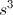, where V is the volume, and s is the length of each side. We can set V to 216 and then solve for s.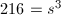In order to find s, we can find the cube root of both sides of the equaton. Finding the cube root of a number is the same as raising that number to the one-third power.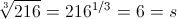This means the length of the side of the cube is 6. We can use this information to find the surface area of the cube, which is equal to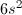. The formula for surface area comes from the fact that each face of the cube has an area of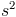, and there are 6 faces in a cube.

surface area =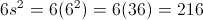The surface area of the square is 216 square units.

### Example Question #5 : How To Find Surface Area

You have a cube with sides of 4.5 inches. What is the surface area of the cube?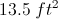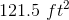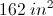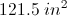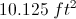Explanation:

The area of one side of the cube is: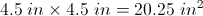A cube has 6 sides, so the total surface area of the cube is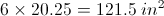### Example Question #1 : How To Find The Surface Area Of A Cube

A cube has a surface area of 24. If we double the height of the cube, what is the volume of the new rectangular box?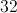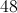Explanation:

We have a cube with a surface area of 24, which means each side has an area of 4. Therefore, the length of each side is 2. If we double the height, the volume becomes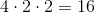.

### Example Question #1 : How To Find The Surface Area Of A Cube

A cube has a surface area of 10m2. If a cube's sides all double in length, what is the new surface area?

80m2

640m2

320m2

40m2

20m2

40m2

Explanation:

The equation for surface area of the original cube is 6s2. If the sides all double in length the new equation is 6(2s)2 or 6 * 4s2. This makes the new surface area 4x that of the old. 4x10 = 40m2

### Example Question #1 : How To Find The Surface Area Of A Cube

What is the surface area of a cube with a side length of 30?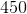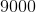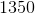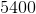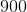Explanation:

Write the formula for the surface area of a cube.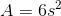Substitute the side.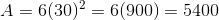### Example Question #3 : How To Find The Surface Area Of A Cube

The surface areas of six cubes form an arithmetic sequence. The two smallest cubes have sidelengths 10 and 12, respectively. Give the surface area of the largest cube.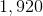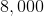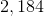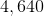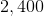Explanation:

The surface area of a cube can be calculated by squaring the sidelength and multiplying by six. The two smallest cubes therefore have surface areas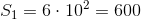and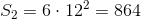The surface areas form an arithmetic sequence with these two surface areas as the first two terms, so their common difference is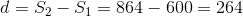.

The surface area of the largest, or sixth-smallest, cube, is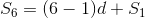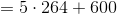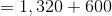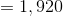← Previous 1